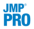Fitting Linear Models
Publication date: 05/24/2021

Fitting Linear Models

# Model Specification

##### Specify Linear Models

Using the Fit Model platform, you can specify complex models efficiently. Your task is simplified by Macros, Attributes, and transformations. Fit Model is your gateway to fitting a broad variety of models and effect structures.

These include:

simple and multiple linear regression

analysis of variance and covariance

random effect, nested effect, mixed effect, repeated measures, and split plot models

nominal and ordinal logistic regression

multivariate analysis of variance (MANOVA)

canonical correlation and discriminant analysis

loglinear variance (to model the mean and the variance)

generalized linear models (GLM)

parametric survival and proportional hazards

response screening, for studying a large number of responsesIn JMP Pro, you can also fit the following:

mixed models with a range of covariance structures

generalized regression models including the elastic net, lasso, and ridge regression

partial least squares

The Fit Model platform lets you fit a large variety of types of models by selecting the desired personality. This chapter focuses on the elements of the Model Specification window that are common to most personalities.